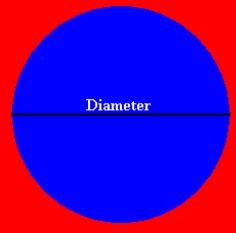# Area and Perimeter of a Circle Calculator

Calculate the Area and Perimeter of a Circle.

The calculation is the same for Metric and Imperial length measurement units and the calculated result is in the unit of your choice.

#### Enter the diameter length of the circle

Select measurement unit:
Diameter of Circle {{selectedunit.m1}}

#### Results:

 Area {{selectedunit.m1}}² Perimeter {{selectedunit.m1}}